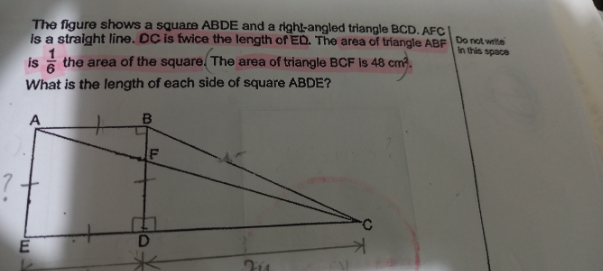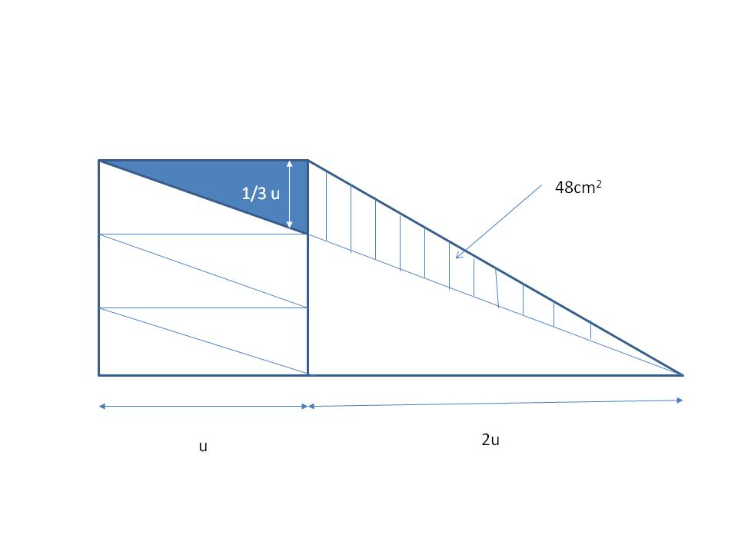# QuestionPrior deductions:

Area of square ABDE effectively = BD x BD

Area of triangle ABC = 1/2 x BD x BD = 1/2 of square ABDE.

Plugging in numerical information,

Area of triangle ABC = Area of triangle ABF + Area of triangle BFC

= 1/6 of square ABDE + 48

1/2 of square ABDE = 1/6 of square ABDE + 48

3/6 of square ABDE = 1/6 of square ABDE + 48

2/6 of square ABDE = 48

6/6 of square ABDE = 48 * 3 = 144

Length of square ABDE = sq root of 144 = 12cm

0 Replies 2 LikesFrom the diagram, using area of triangle  1/2 b x h on the triangle on the right with stripes,

(1/2)(1/3)u (2u) = 48

1/3 u2 = 48

u2  = 48 x 3 = 144

u = 12

Hence the length of each side of square is 12cm.

0 Replies 2 Likes